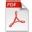# Math Course Syllabi

 Course Number and Name PDF MS Word MA 100 - Intermediate AlgebraMA 100E - Intermediate AlgebraMA 105 - Introduction to Finite MathematicsMA 110 - Finite MathematicsMA 111 - Mathematical Reasoning for the ArtsMA 112 - College AlgebraMA 113 - College TrigonometryMA 115 - Pre-calculus Algebra & TrigonometryMA 121 - Calculus for Business and Life Sciences IMA 122 - Calculus for Business and Life Sciences IIMA 125 - Calculus IMA 126 - Calculus IIMA 147 - Elementary StatisticsMA 227 - Calculus IIIMA 237 - Linear AlgebraMA 238 - Applied Differential EquationsMA 306 - Mathematics for the Elementary School TeacherMA 421 - College GeometryMA 425 - Methods & Materials for Teaching Secondary Mathematics